# SAT II Math II : Range

## Example Questions

### Example Question #1 : Range

Find the range of the following data set: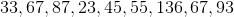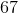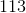Explanation:

Find the range of the following data set:To find the range, find the difference between the largest and smallest values.

Largest: 136

Smallest:23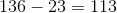So our range is 113

### Example Question #2 : Range

Determine the range of the numbers.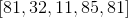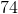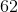Explanation:

The range of the numbers is the difference between the largest and smallest numbers.

The largest number is 85.

The smallest number is 11.

Subtract both numbers.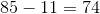The answer is:### Example Question #3 : Range

Determine the range of the numbers: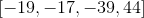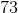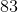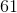Explanation:

To find the range, subtract the highest with the lowest number provided in the set of numbers.

The highest number is 44.

The smallest number is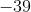.

Subtract both quantities.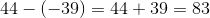The answer is:### Example Question #4 : Range

Identify the range of the numbers: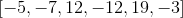Explanation:

The range is the difference between the largest and smallest numbers.

The largest number is:The smallest number is:Subtract both numbers.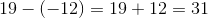The answer is:### Example Question #5 : Range

Determine the range of the numbers: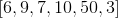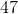Explanation:

The range is the difference between the largest and smallest numbers.

The largest number is 50, and the smallest number is 3.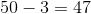The answer is:### Example Question #6 : Range

Find the range given the following numbers: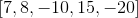Explanation:

The range is the difference between the largest and smallest numbers.

The largest number is 15.

The smallest number is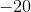.

Subtract the two numbers.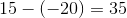The answer is:### Example Question #7 : Range

Determine the range: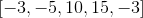Explanation:

The range is the difference between the largest and smallest numbers.

The largest number is 15.

The smallest number is -5.

Subtract both numbers.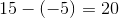The answer is.